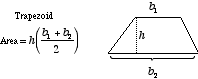index: click on a letter A B C D E F G H I J K L M N O P Q R S T U V W X Y Z A to Z index index: subject areas numbers & symbols sets, logic, proofs geometry algebra trigonometry advanced algebra & pre-calculus calculus advanced topics probability & statistics real world applications multimedia entrieswww.mathwords.com about mathwords website feedback

 Trapezoid US usage, definition 1: A quadrilateral which has a pair of opposite sides which are parallel. The parallel sides are called the bases, and the other two sides are called the legs. US usage, definition 2: A quadrilateral which has one parallel pair of opposite sides and one non-parallel pair of opposite sides. The parallel sides are called the bases, and the other two sides are called the legs. UK usage: The same as the US word trapezium. The UK word trapezium means the same as the US word trapezoid, and vice-versa.Commentary: Under US definition 1, a parallelogram is a type of trapezoid. Under US definition 2, a parallelogram is not a type of trapezoid. Regardless of which definition you prefer, the trapezoid area formula can be used to find the area of a parallelogram.   See also